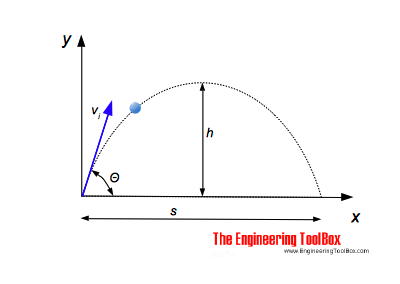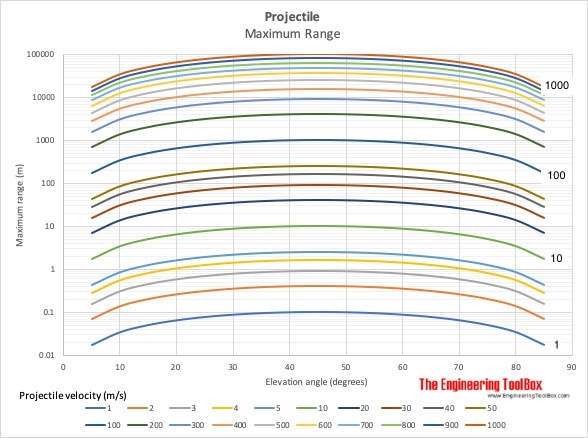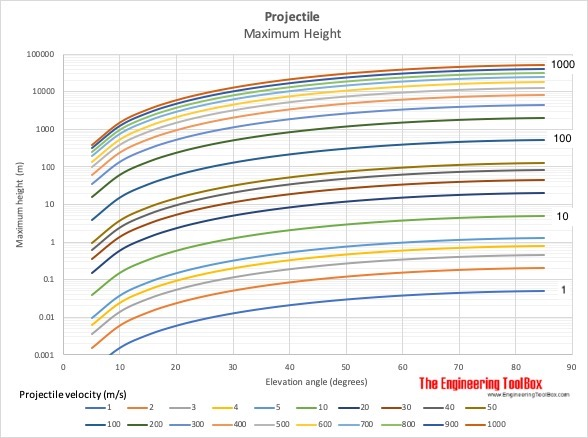Engineering ToolBox - Resources, Tools and Basic Information for Engineering and Design of Technical Applications!

# Projectile Range

## Calculate the range of a projectile - a motion in two dimensions.The time for a projectile - a bullet, a ball or a stone or something similar - thrown out with an angle Θ to the horizontal plane - to reach the maximum height can be calculated as

th = vi sin(Θ) / ag                                 (1)

where

th = time to reach maximum height (s)

vi = initial velocity of the projectile (m/s, ft/s)

Θ = the initial angle of the velocity vector to the horizontal plane (degrees)

ag = acceleration of gravity (9.81 m/s232.174 ft/s2)

The total flight time can be expressed as

ts = 2 th

= 2 vi sin(Θ) / ag                      (2)

where

ts = time for the total flight (s)

The maximum elevation - h - of the flight can be calculated  as

h = 1/2 ag th2                              (3)

where

h = flight maximum elevation (m, ft)

The horizontal distance of the flight can be expressed as

s = vi2 sin(2 Θ) / ag                           (4)

where

s = flight distance (m, ft)

### Example - Throwing a Ball

A ball is thrown with initial velocity 25 m/s in angle 30 degrees to the horizontal plane. The time for the ball to reach maximum level is

th = (25 m/s) sin(30 degrees) / (9.81 m/s2)

= 1.27

The maximum elevation of the ball can be calculated as

h = 1/2 (9.81 m/s2) (1.27 s)2

= 7.91 m

The horizontal distance traveled by the ball can be calculated as

s = (25 m/s)2 sin(2 30 degrees) / (9.81 m/s2)

= 55.2 m

### Projectile Flight Calculator

The calculator below can be used to estimate the time of flight, time to reach maximum elevation, maximum elevation and flight distance for a projectile like a bullet or a ball or something similar.

Note that friction force due to air resistance is neglected.

vi - initial velocity (m/s)

Θ - initial angle to the horizontal plane (degrees)Note that friction force due to air resistance is neglected in the charts above. For higher velocities range and maximum height will be dramatically reduced.

## Related Topics

• ### Dynamics

Motion - velocity and acceleration, forces and torque.
• ### Mechanics

Forces, acceleration, displacement, vectors, motion, momentum, energy of objects and more.

## Related Documents

• ### Acceleration of Gravity and Newton's Second Law

Acceleration of gravity and Newton's Second Law - SI and Imperial units.
• ### Angular Motion - Power and Torque

Angular velocity and acceleration vs. power and torque.
• ### Centripetal and Centrifugal Acceleration Force

Forces due to circular motion and centripetal / centrifugal acceleration.
• ### Conservation of Momentum

The momentum of a body is the product of its mass and velocity - recoil calculator.
• ### Distance Traveled vs. Speed and Time - Calculator and Chart

Velocity plotted in time used diagram.
• ### Formulas of Motion - Linear and Circular

Linear and angular (rotation) acceleration, velocity, speed and distance.
• ### Impulse and Impulse Force

Forces acting a very short time are called impulse forces.
• ### Kinetic Energy

Energy possessed by an object's motion is kinetic energy.
• ### Satellites in Orbit

Orbital and escape velocity for geostationary satellites.

## Engineering ToolBox - SketchUp Extension - Online 3D modeling!

Add standard and customized parametric components - like flange beams, lumbers, piping, stairs and more - to your Sketchup model with the Engineering ToolBox - SketchUp Extension - enabled for use with older versions of the amazing SketchUp Make and the newer "up to date" SketchUp Pro . Add the Engineering ToolBox extension to your SketchUp Make/Pro from the Extension Warehouse !

We don't collect information from our users. More about

## Citation

• The Engineering ToolBox (2014). Projectile Range. [online] Available at: https://www.engineeringtoolbox.com/range-projectile-d_1908.html [Accessed Day Month Year].

Modify the access date according your visit.

9.19.12